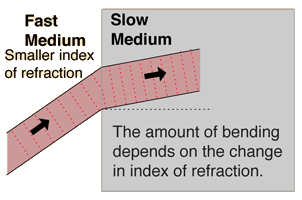# Question f8fdc

$v = \frac{c}{n}$
Where $v$ is the velocity of light in the medium, $c$ (~#3.0x${10}^{8}$ m/s) is the speed of light in vacuum, and $n$ is the index of refraction of the medium.